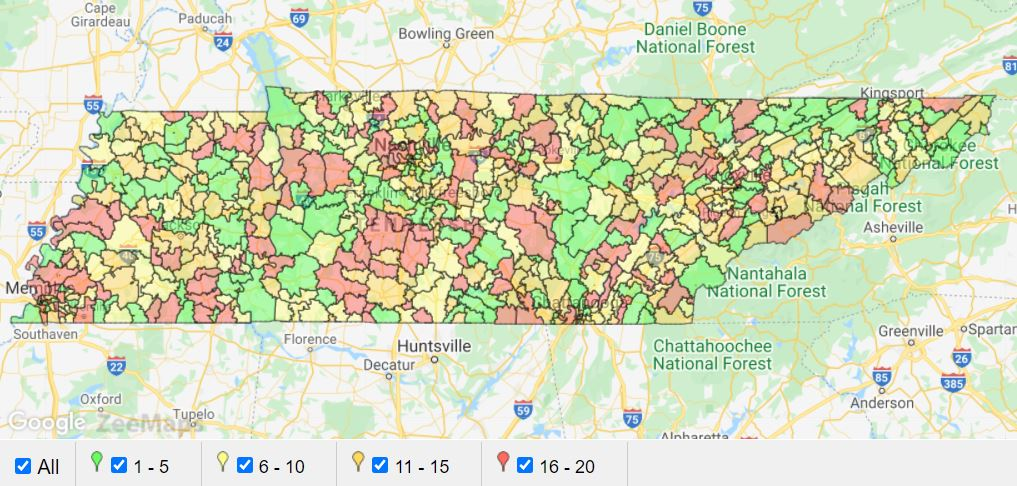## IF Function in Excel – IFS Function in ZeeMaps

A type of IF function in Excel is the IFS function in which you can input multiple conditions to determine cell values. The function is checked from left to right for the multiple conditions, and the value set for the cell is determined by the first condition that is met. IFS is a much easier way of testing for multiple conditions instead of nesting multiple IF functions.

This statement is extremely helpful when we want to make one field value dependent on another. For example, if the score is => 80, then the Grade is B; if the score is =>90, then Grade is A.

In this post we will show you how to assign different colors to pins or highlighted regions when you upload a spreadsheet. We will use a spreadsheet that has zip codes in the US with a column for number of dealers in that zip code. The sample spreadsheet is attached to the bottom of this post and has just two columns, ‘zip code’ and ‘dealers’. We’ll add a third column – ‘colors’.

## Find the Color Name

First, for this task we need to take a look at the color options in ZeeMaps, which are listed here. All ZeeMaps users have access to 32 colors and users on our Enterprise plan have access to 72 colors. To use the extended color palette, please see our blog post.

Color Name for Excel IF Function

For our IF Function, we will need to assign different color values based on values in a given column of our spreadsheet, e.g., number of dealers, grades, etc. For this purpose, note the Color Name in the color list. Use this as the color value in your expression.

## How to write the Excel IF Function

Generally, the syntax for the Excel IFS function is:

=IFS([Something is True1, Value if True1,Something is True2,Value if True2,Something is True3,Value if True3)

The Excel IF Function allows you to test up to 127 different conditions.

Note that the conditions need to be entered in the correct order, and can be very difficult to build, test and update if you have entered a large number of conditions.

### The Expression – Excel IF Function

We entered the following Excel IF Function expression for our example below. Since our spreadsheet column B contains the number of dealers, we’ll enter an IF Function expression in cell C2 as follows:

=IFS(B2 <= 5, “Green”, B2 <= 10, “Light Yellow”, B2 <= 15, “Yellow”, B2 <= 20, “Red”)